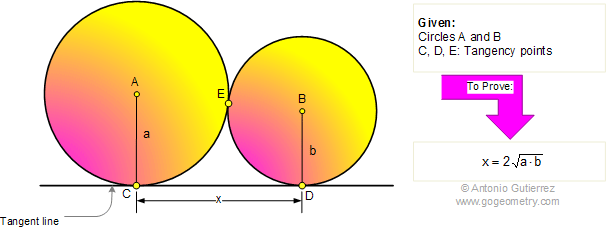# Problem 314: Tangent circles, Common external tangent line, Geometric Mean

 The figure shows circles A (radius a) and B (radius b) tangent at E. A common tangent line is tangent to the circles at C and D, respectively. Prove that CD is twice the geometric mean of a and b. View or Post a solution.